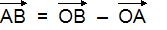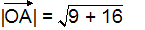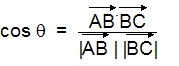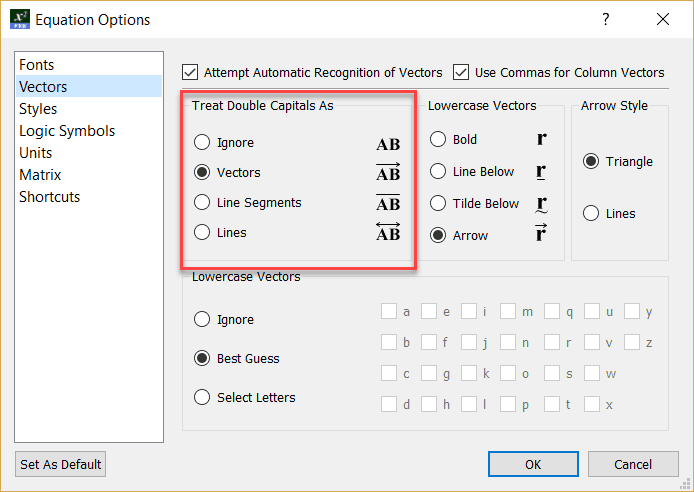﻿ Double Capitals (COPY)

# Double Capitals

Top  Previous  Next

FX Equation technology will only detect Double Capital vectors if automatic recognition is switched on.

Double capital letters are used to represent line segments, rays and vectors.  The equation tool can automatically place an annotation over double capitals.  You would use this feature if you were, for example, entering a lot of position vectors in an exam.

## Examples:is entered as AB = OB - OAis entered as |OA|=sr(9+16)is entered as cos th = (AB.BC)/(|AB | |BC|)

The annotation used by the equation tool can be set using the Vector Options